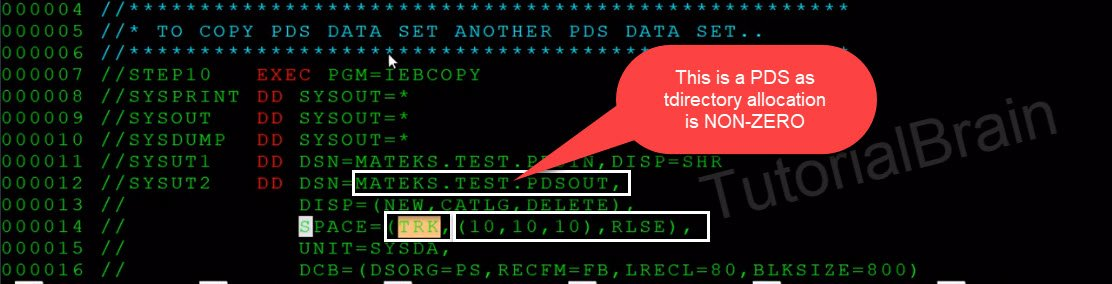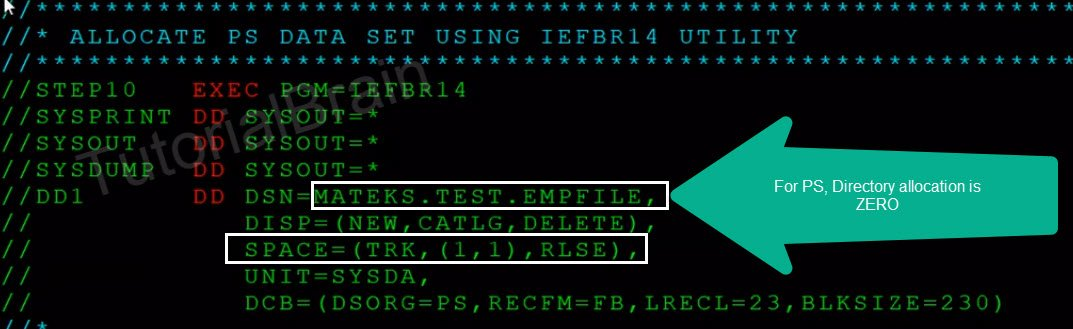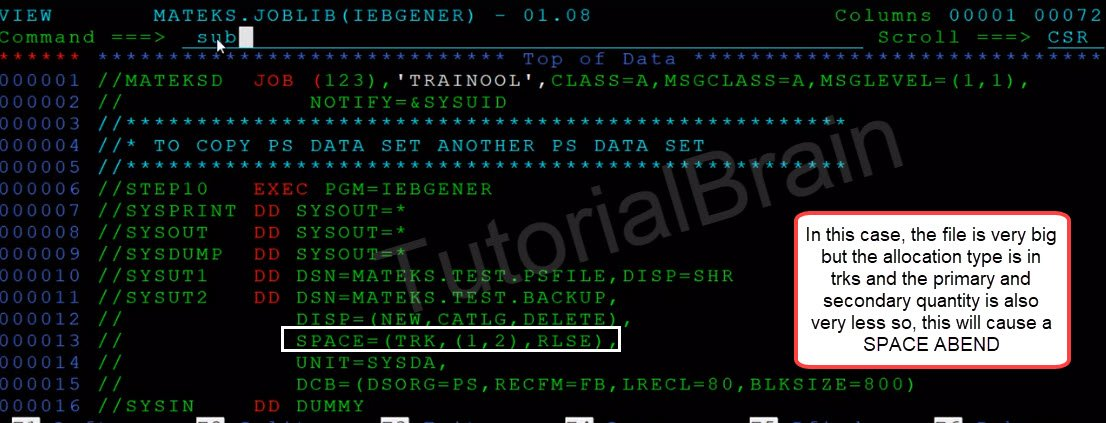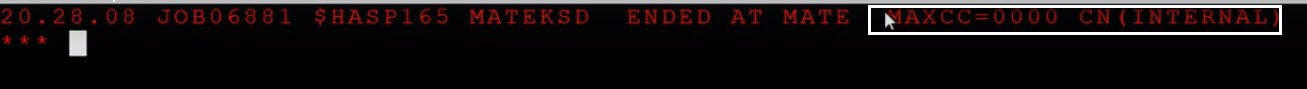# JCL SPACE Parameter

## JCL SPACE Parameter

JCL SPACE Parameter is an optional Keyword parameter and it is used to provide the space-related details for the dataset.

SPACE Parameter in JCL is one of the most important parameters in JCL which is a way to tell the JCL that what is the amount of storage which you want to provide for your dataset and what is the allocation type of the storage?

###### Syntax:
SPACE=(Space_aloc_type,(Primary_qnty,Secondary_qnty,Drtry)[,RLSE][,CONTIG][,MXIG][,ROUND])

a) Space_aloc_type – Space allocation type is the unit of measurement which will take any of these values:

• TRK
• CYL
• BLKLGTH
• RECLGTH

b) Primary_qnty – Primary Quantity – We need to provide the minimum value of primary space required for the dataset.

###### c) Secondary_qnty –

Secondary Quantity – We need to define a value of Secondary quantity because when it fills the amount of primary space, it requires a secondary quantity where it is the additional space and the system provides to the resources used in the step

###### d) Drtry

– This is used for the Directory. You need to decide on how many members you should have in the PDS and based on that you need to give the value of the directory. For the PS file, the value of the directory is always 0 because PS does not contain any members.

###### e) RLSE

– Means the memory which is allocated to this dataset needs to be released, once this dataset is closed
f) CONTIG – Allocate the memory in a contiguous location
g) MXIG – Allocate the memory to the largest contiguous memory location
h) ROUND
Used when average block length is allocated. It is used to request for an entire cylinder for storage of the data set

The Most common way to defining SPACE Parameter:
TRK,
SPACE=( CYL, (prim-allocation[,sec-allocation][,directory) )
blksize,

The above SPACE Parameter has two positional sub-parameters.
1) Unit of measurement type – Most used values is TRK, CYL or BLKSIZE. The RECLGTH(i.e. the value of record length) is also used in few cases.
2) primary, secondary and directory – prim-allocation[,sec-allocation][,directory)

###### Note/warning/suggestion/improvement:

1) Directory allocation is only for PDS and PS, the value is always ZERO.
2) Give enough space allocation to avoid space, Abend.
If primary and secondary spaces are exhausts, the system throws an Abend-
SB37 Abend – For PS dataset
SE37 Abend – For PDS
Sometimes, it gives S013-14 abend as well
3) Code proper values for primary allocation. Secondary and directory space if omitted defaults to zero.

## Sample Example-1 for SPACE Parameter## Explanation of SPACE=(TRK,(10,10,10),RLSE)

This means as the allocation must happen in Tracks.

The Primary Quantity is 10 and if this primary quantity is exhausted that means if the value of 10 is exhausted then allocate this secondary quantity of 10.

The directory is 10 because in this case, we want to create a PDS so we need to define a PDS so you need to give a Non-Zero value for the directory block and we have given this value as 10.

If we need to create a PS, then it is not required to code any value for the directory because the value is always 0 for PS.

RLSE tells the System to release all the memory resources for this particular PDS.

## Sample Example-2 for SPACE Parameter## Explanation of SPACE=(TRK,(1,1),RLSE)

This means like the allocation must happen in Tracks.

The Primary Quantity is 1 and if it exhausts this primary quantity then the system should allocate this secondary quantity of 1. It will not define directory because in this case, we want to create a PS.

RLSE will tell the system to release the resources which were allocated for this PS file when the files are closed.

## Let us see if we code improper value of SPACE Parameter

Suppose, we have a BIG file and we need to copy this file to a new file and if we define the SPACE Parameter of the new file as very less, then it will throw a SPACE ABEND. Let us see how –This input file is BIG and it contains 211666 lines of data as shown above –We have coded a very less value for the SPACE Parameter as shown above which is SPACE=(TRK,(1,2),RLSE)

## Output in this case -You can see that the JOB throws a SPACE ABEND which is SB37 Abend

## Let us see if we code a proper SPACE Parameter

Suppose, we have the same input file as shown in above where it is caused the SB37 Abend. Then, we can fix this by providing a good amount of SPACE allocation for the output file which needs to be created as shown below-We have provided a good amount of space for the output file as shown above –

## Output in this case -You can see that the JOB completes successfully without any issues.